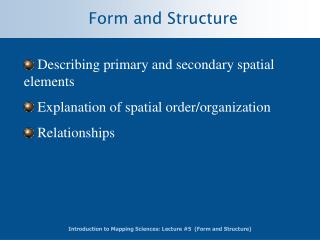# Form and Structure - PowerPoint PPT PresentationDownload PresentationForm and Structure

Form and StructureDownload Presentation## Form and Structure

- - - - - - - - - - - - - - - - - - - - - - - - - - - E N D - - - - - - - - - - - - - - - - - - - - - - - - - - -
##### Presentation Transcript

1. Form and Structure • Describing primary and secondary spatial elements • Explanation of spatial order/organization • Relationships

2. Form and Structure • Edge • Shape • Orientation • Composition • Arrangement • Connectivity • Trends & Cycles • Hierarchy/Order

3. Edge • Boundary • Distinction between two features • Change in identity

4. Shape • The geometric form of a feature • Empirical shape vs. Standard shape

5. Shape Compactness • Comparison of Area to Perimeter • Shape Index • SI = 2(A/2.82(P) • Circle = 1

6. Shape Distortion • Function of Projection and coordinate system • Example is Mercator

7. 3-D Shape • Profiles • Profiles are used to take cross-sections of three dimensions. • They are particularly effective to represent terrain

8. Orientation • Direction

9. Composition • HomogeneityThe consistent dispersion of a single feature. Uniformity can occur in size, shape, orientation, dispersion, connectivity etc. • Diversity (heterogeneity)A mixture of features (e.g. biodiveristy). Can apply to housing, agriculture forests etc. • CommunityDiversity with a strong component among the assemblage of features. Ecologist often talk about "plant communities" and urban planners about"sense of community".

10. Arrangement • Dispersion • Spacing

11. Terminology Clustered Scattered Random

12. Measures of Central Tendency • Mean Center • Weighted Mean Center • Median Center

13. Mean Center • Similar to arithmetic mean, only with two coordinates

14. Weighted Mean Center • Uses weights to ‘shift’ mean center

15. Example

16. Density Based Measures • Quadrat Analysis • Density Estimation

17. Overview of Quadrat Analysis Overlay empty grid on distribution of points Count frequency of points within each grid cell Calculate the mean and variance of frequencies within grid cells Calculate the variance to mean ratio to determine amount of clustering Variance/mean ratio values significantly greater than 1 suggest a clustered pattern Test for statistical significance

18. Quadrat Summary 0 0 0 0 0 0 0 0 0 0 + 0 0 0 0 0 0 0 0 0 0 0 0 0 0 0 Empty Grid Map of Incident Locations 0 2 3 6 3 0 1 4 6 4 = 4 3 9 8 5 3 5 7 6 3 2 3 5 4 2 Image of Incident Frequencies

19. Density • Density estimation measures densities in a grid based on a distribution of points and point values. • A simple density estimation method is to place a grid on a point distribution, tabulate points that fall within each cell, sum the point values, and estimate the cell's density by dividing the total point value by the cell size. • A circle, rectangle, wedge, or ring based at the center of a cell may replace the cell in the calculation.

20. Visual Kernel Estimation

21. Kernel Estimation

22. Kernel Estimation

23. Distance Based Measures • Euclidean Distance • Nearest Neighbor Distance • (Clark and Evans, 1954)

24. Nearest Neighbor Index • Expected Nearest Neighbor Distance A: area where points distribute n: number of points • Nearest Neighbor Index If the actual points are randomly distributed, DA should be close to DE, thus NNI is close to unity. However, if the points are clustered, DA would be close to zero, and so is NNI. The more scattered the points are distributed, the larger the distance between points and NNI reaches its maximum at 2.1491.

25. Connectivity • Linkages • ‘Distances’

26. Connectivity A/3(n-2)

27. Connectivity A/n(n-1)/2

28. Connectivity A/n(n-1)

29. Connectivity B C A E D

30. Connectivity via Matrices A B C D E A B C D E 0 1 0 0 1 1 0 1 0 1 0 1 0 1 0 0 0 1 0 1 1 1 0 1 0

31. Trends and Cycles • Trend is the tendency of a feature to increase or decrease. • Some trends are physically observable (landforms, people density on subway) others need to be experienced (temperature gradient up a mountain). • Some of the more simpler trends can be characterized with the terms constant, convex, concave to describe ground surface profiles and dome, plunging ridge, or saddle to describe terrain. • Cyclical phenomena have a repetitive character and can be described mathematically for two and three dimensional features.

32. Hierarchy and Order • Hierarchies are usually created as way of showing the importance of different components of a system. • For instance stream segments which have no tributaries are said to be first order streams. Second order have 1 tributary etc.